# xticks

Set or query x-axis tick values

## Syntax

xticks(ticks)
xt = xticks
xticks('auto')
xticks('manual')
m = xticks('mode')
___ = xticks(ax,___)

## Description

example

xticks(ticks) sets the x-axis tick values, which are the locations along the x-axis where the tick marks appear. Specify ticks as a vector of increasing values; for example, [0 2 4 6]. This command affects the current axes.
xt = xticks returns the current x-axis tick values as a vector.

example

xticks('auto') sets an automatic mode, enabling the axes to determine the x-axis tick values. Use this option if you change the tick values and then want to set them back to the default values. 
xticks('manual') sets a manual mode, freezing the x-axis tick values at the current values. Use this option if you want to retain the current tick values when resizing the axes or adding new data to the axes.
m = xticks('mode') returns the current x-axis tick values mode, which is either 'auto' or 'manual'. By default, the mode is automatic unless you specify tick values or change the mode to manual.

example

___ = xticks(ax,___) uses the axes specified by ax instead of the current axes. Specify ax as the first input argument for any of the previous syntaxes.

## Examples

collapse all

Create a line plot. Display tick marks along the x-axis at the values 0, 5, and 10. Then specify a label for each tick mark.

x = linspace(0,10); y = x.^2; plot(x,y) xticks([0 5 10]) xticklabels({'x = 0','x = 5','x = 10'})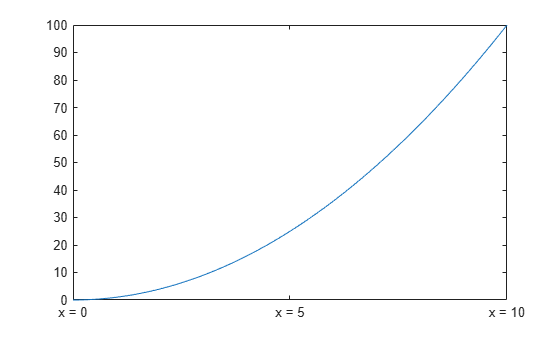Display tick marks along the x-axis at nonuniform values between -5 and 5. MATLAB® labels the tick marks with the numeric values.

x = linspace(-5,5); y = x.^2; plot(x,y) xticks([-5 -2.5 -1 0 1 2.5 5])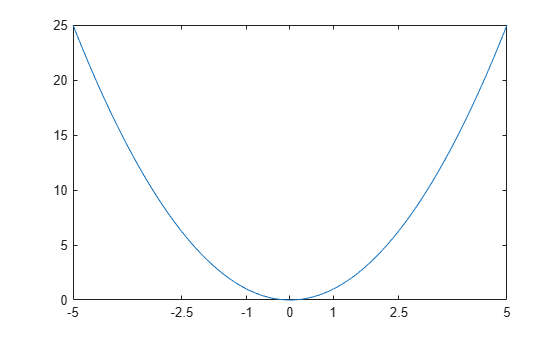Display tick marks along the x-axis at increments of 10, starting from 0 and ending at 50.

x = linspace(0,50); y = sin(x/2); plot(x,y) xticks(0:10:50)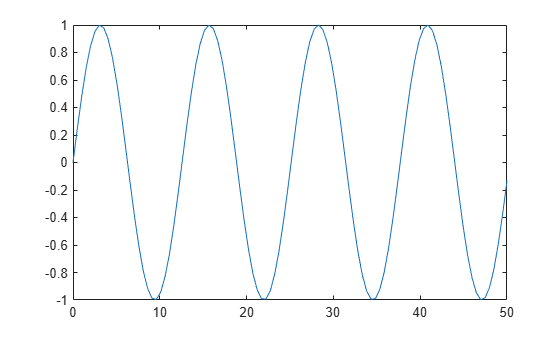Create a line plot. Specify the x-axis limits as 0 to $6\pi$. Then, display tick marks along the x-axis at increments of $\pi$.

x = linspace(0,6*pi); y = sin(x); plot(x,y) xlim([0 6*pi]) xticks(0:pi:6*pi)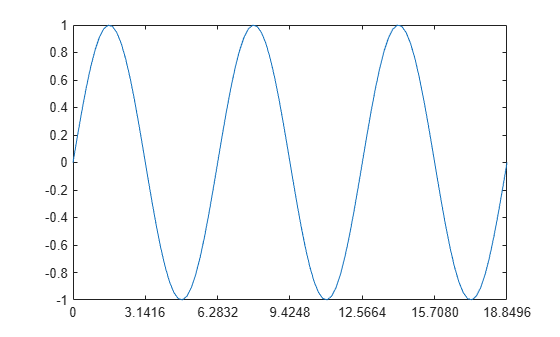MATLAB® labels the tick marks with the numeric values. Change the labels to show the $\pi$ symbol by specifying the text for each label.

xticklabels({'0','\pi','2\pi','3\pi','4\pi','5\pi','6\pi'})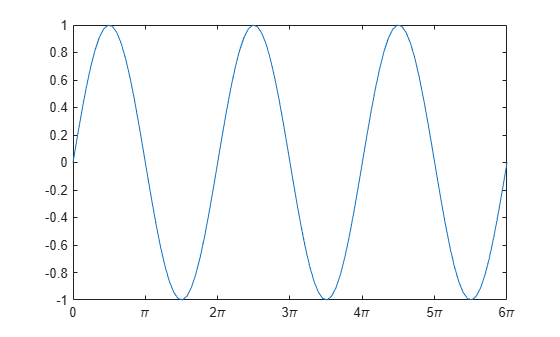Create a plot with duration values along the x-axis. Then, change the duration values where the tick marks are located.

t = minutes(0:.5:3); y = rand(1,7); plot(t,y)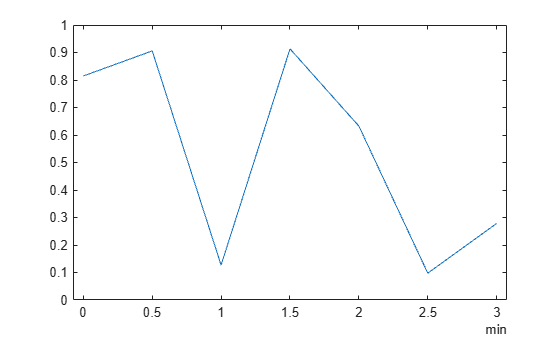ticks = minutes(0:.25:3); xticks(ticks)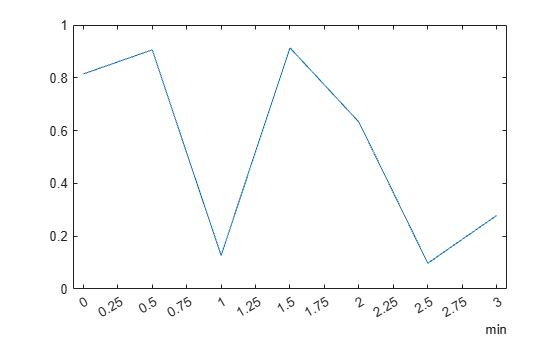Create a stem chart and specify the x-axis tick values. Then, set the x-axis tick values back to the default values.

stem(1:10) xticks([0 4 6 10])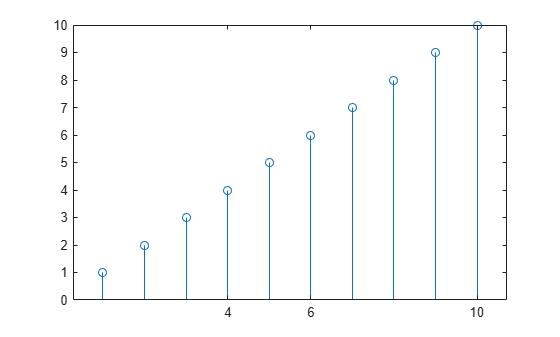xticks('auto')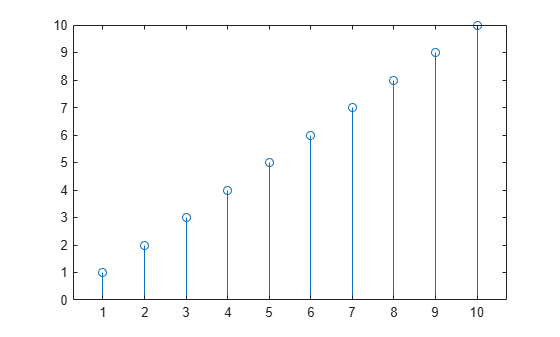Starting in R2019b, you can display a tiling of plots using the tiledlayout and nexttile functions. Call the tiledlayout function to create a 2-by-1 tiled chart layout. Call the nexttile function to create the axes objects ax1 and ax2. Plot random data into each axes. Then set the x-axis tick values for the lower plot by passing ax2 as the first input argument to the xticks function.

tiledlayout(2,1) ax1 = nexttile; plot(rand(3)) ax2 = nexttile; plot(rand(3)) xticks(ax2,[1 2 3])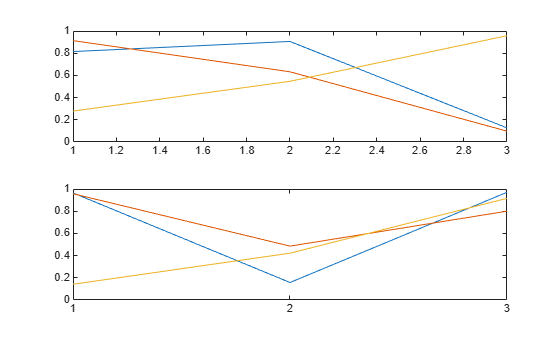Remove the tick marks along the x-axis by specifying the tick values as an empty array.

x = linspace(0,10); y = sin(x); plot(x,y) xticks([])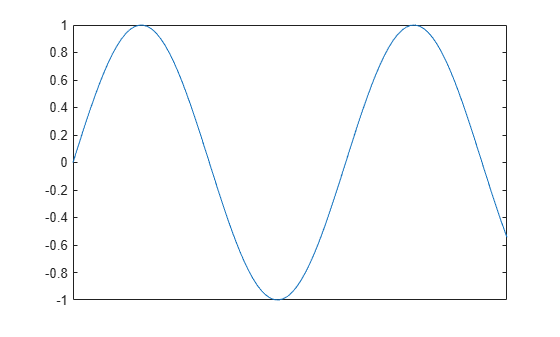## Input Arguments

collapse all

Tick values, specified as a vector of increasing values. If you do not want tick marks along the x-axis, specify an empty vector [].

You can specify the tick values as numeric, categorical, datetime, or duration values. However, the type of values that you specify must match the type of values along the x-axis.

Example: xticks([pi 2*pi 3*pi 4*pi])

Example: xticks(0:10:100)

Example: xticks([])

Note

To specify the tick labels, use the xticklabels function.

Data Types: single | double | int8 | int16 | int32 | int64 | uint8 | uint16 | uint32 | uint64 | categorical | datetime | duration

Target axes, specified as an Axes object or an array of Axes objects.

If you do not specify this argument, then xticks modifies the current axes.

## Output Arguments

collapse all

Current tick values, returned as a vector.

Current mode, returned as one of these values:

• 'auto' — Automatically determine the x-axis tick values.

• 'manual' — Use manually specified x-axis tick values.

collapse all

### Tick Values

The tick values are the locations along the x-axis where the tick marks appear. The tick labels are the labels that you see for each tick mark. Set the values using the xticks function. Set the corresponding labels using the xticklabels function.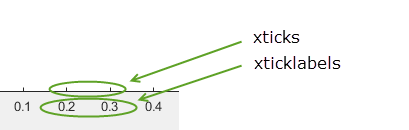## Algorithms

The xticks function sets and queries several axes properties related to the x-axis tick values.

• XTick — Property that stores the x-axis tick values.

• XTickMode — Property that stores the x-axis tick value mode. When you set the x-axis tick values, this property changes to 'manual'.

## Version History

Introduced in R2016b